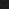JavaPerspective.com  >   Beginner Tutorials  >   4. Object-Oriented Concepts  >   4.2. Constructors

4.2. Constructors
Last updated: 23 January 2013.

A constructor is a method that is called when a new instance of a class is created. In fact, the keyword new calls, if any, the constructor of the object it is creating. A constructor's signature looks like this:

public <className> ( [<parameters>] ) {

// the constructor's code goes here
}

The parameters are optional and the constructor does not have a return type. As an example, let's add a constructor to the class Aircraft:

public class Aircraft {

float length;

float height;

int range;

double weight;

boolean airborne;

public Aircraft(){

// the constructor's code goes here

}

public boolean land(){

if(airborne == true){

airborne = false;

return true;

}

return false;

}

public boolean takeOff(){

if(airborne == false){

airborne = true;

return true;

}

return false;

}

}

Basically, a constructor is used to run the code that you want to be executed in the first place when the object is created. For example, you may want to initialize certain variables of the class Aircraft at creation time. In that case, the constructor can take parameters corresponding to the variables that are to be initialized:

public class Aircraft {

float length;

float height;

int range;

double weight;

boolean airborne;

public Aircraft(float length, float height, int range, double weight){

this.length = length;

this.height = height;

this.range = range;

this.weight = weight;

}

public boolean land(){

if(airborne == true){

airborne = false;

return true;

}

return false;

}

public boolean takeOff(){

if(airborne == false){

airborne = true;

return true;

}

return false;

}

}

The keyword this is used within a class to reference the current object. In the example shown above, the keyword this references the current instance of the class Aircraft. The keyword this can access all the fields and methods of the object in which it is used.

The line of code below creates a new instance of the class Aircraft with initialization values for its length, height, range and weight:

Aircraft aircraft = new Aircraft(58.17F, 12.7F, 257, 17650.5D);

A class can have multiple constructors. In that case, each constructor must have a unique signature. For example, if you also want to be able to initialize the variable airborne when the object is created, you can add another constructor to the class Aircraft:

public Aircraft(float length, float height, int range, double weight, boolean airborne){

this.length = length;

this.height = height;

this.range = range;

this.weight = weight;

this.airborne = airborne;
}

Finally, the class Aircraft has 3 constructors (if I include the very first constructor that did not have any parameters), allowing 3 different ways of creating an Aircraft object:

public class Aircraft {

float length;

float height;

int range;

double weight;

boolean airborne;

public Aircraft(){

}

public Aircraft(float length, float height, int range, double weight){

this.length = length;

this.height = height;

this.range = range;

this.weight = weight;

}

public Aircraft(float length, float height, int range, double weight, boolean airborne){

this.length = length;

this.height = height;

this.range = range;

this.weight = weight;

this.airborne = airborne;

}

public boolean land(){

if(airborne == true){

airborne = false;

return true;

}

return false;

}

public boolean takeOff(){

if(airborne == false){

airborne = true;

return true;

}

return false;

}

}

Last but not least, you may sometimes need to execute the same statements in all the constructors. For example, let's say you want to print the message Aircraft created when a new Aircraft instance is created. To that end, the following statement has to be included in all the constructors:

System.out.println("Aircraft created");

In order to avoid repeating the same statement in all the constructors, you can use an initialization block:

public class Aircraft {

float length;

float height;

int range;

double weight;

boolean airborne;

// This is an initialization block

{

System.out.println("Aircraft created");

}

public Aircraft(){

}

public Aircraft(float length, float height, int range, double weight){

this.length = length;

this.height = height;

this.range = range;

this.weight = weight;

}

public Aircraft(float length, float height, int range, double weight, boolean airborne){

this.length = length;

this.height = height;

this.range = range;

this.weight = weight;

this.airborne = airborne;

}

public boolean land(){

if(airborne == true){

airborne = false;

return true;

}

return false;

}

public boolean takeOff(){

if(airborne == false){

airborne = true;

return true;

}

return false;

}

}

As you can see, an initialization block starts with an opening brace and ends with a closing brace. The statements in the initialization block are automatically included in all the constructors. As an example, you can run the following statements in a main method:

Aircraft aircraft1 = new Aircraft();
Aircraft aircraft2 =
new Aircraft(58.17F, 12.7F, 257, 17650.5D);
Aircraft aircraft3 =
new Aircraft(58.17F, 12.7F, 257, 17650.5D, false);

The output is:

Aircraft created
Aircraft created
Aircraft created

You are here :  JavaPerspective.com  >   Beginner Tutorials  >   4. Object-Oriented Concepts  >   4.2. Constructors
Next tutorial :  JavaPerspective.com  >   Beginner Tutorials  >   4. Object-Oriented Concepts  >   4.3. Static members